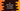# Find the seconds and milliseconds time difference between two time in C### C# program to find the time difference between two dates in seconds:

Sometimes, we need to calculate the time difference in seconds. For example, if you are writing a game, the time difference even in milliseconds matterns a lot. In this post, we will learn how to find the time difference between two dates in seconds and also in milliseconds.

Finding the time difference and to calculate it in different format like seconds, milliseconds etc. is easy. We already have all the methods given. We just need to know how to use them.

DateTime structure is responsible for dealing with time in C#.

Let’s see how to do that with examples.

## Time difference of two DateTime Objects:

To find the time difference of two DateTime objects, we can use Subtract method or simply -. The difference gives one TimeSpan object. This object provides different properties to convert the value to seconds and milliseconds.

Following are the properties in a TimeSpan object to read the time in different format:

• TotalDays: It gives the time in days
• TotalHours: It gives the time in hours
• TotalMinutes: It gives the time in minutes
• TotalSeconds: It gives the time in seconds
• TotalMilliseconds: It gives the time in milliseconds

We need to use TotalSeconds and TotalMilliseconds to get the time difference in seconds and milliseconds.

### C# program:

Let’s write down the complete program:

``````using System;

public class Program
{
public static void Main()
{
DateTime firstDate = DateTime.Now;

TimeSpan dateDifference = secondDate.Subtract(firstDate);

Console.WriteLine("Difference in milliseconds :"+dateDifference.TotalMilliseconds);
Console.WriteLine("Difference in seconds :"+dateDifference.TotalSeconds);
}
}``````

Here,

• firstDate is holding the first date, which is the current time.
• secondDate is the time after 5 minutes. We are using AddMinutes method to add 5 minutes to the current time.
• The difference between both times is calculated by using the Subtract method. This method finds the difference between firstDate and secondDate.
• The last two lines are printing the total milliseconds and total seconds. Here, we are using TotalMilliseconds and TotalSeconds properties of dateDifference. dateDifference is a TimeSpan object.

It will print the below output:

``````Difference in milliseconds :300000
Difference in seconds :300``````

Instead of Subtract, we can also use - as like below:

``````using System;

public class Program
{
public static void Main()
{
DateTime firstDate = DateTime.Now;